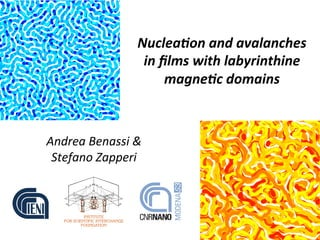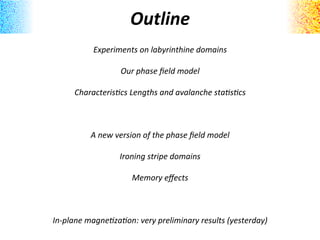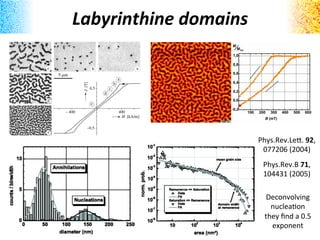Diese Präsentation wurde erfolgreich gemeldet.

# Nucleation and avalanches in film with labyrintine magnetic domains

Anzeige
Anzeige
Anzeige
Anzeige
Anzeige
Anzeige
Anzeige
Anzeige
Anzeige
Anzeige
Anzeige×

1 von 22 Anzeige

# Nucleation and avalanches in film with labyrintine magnetic domains

Experimental investigations of the scaling behavior of Barkhausen avalanches in out-of plane ferromagnetic films yield widely different results for the values of the critical exponents despite similar labyrinthine domain structures, suggesting that universality may not hold for this class of materials. Analyzing a phase-field model for magnetic reversal, we show that avalanche scaling is bounded by characteristic length scales arising from the competition between dipolar forces and exchange interactions. We compare our results with the experiments and find a good qualitative and quantitative agreement, reconciling apparent contradictions. Finally, we make some prediction, amenable to experimental verification, on the dependence of the avalanche's behavior from the film thickness and disorder.

Experimental investigations of the scaling behavior of Barkhausen avalanches in out-of plane ferromagnetic films yield widely different results for the values of the critical exponents despite similar labyrinthine domain structures, suggesting that universality may not hold for this class of materials. Analyzing a phase-field model for magnetic reversal, we show that avalanche scaling is bounded by characteristic length scales arising from the competition between dipolar forces and exchange interactions. We compare our results with the experiments and find a good qualitative and quantitative agreement, reconciling apparent contradictions. Finally, we make some prediction, amenable to experimental verification, on the dependence of the avalanche's behavior from the film thickness and disorder.

Anzeige
Anzeige

### Nucleation and avalanches in film with labyrintine magnetic domains

1. 1. Nuclea'on  and  avalanches     in  ﬁlms  with  labyrinthine   magne'c  domains   Andrea  Benassi  &   Stefano  Zapperi
2. 2. Outline   Experiments  on  labyrinthine  domains     Our  phase  ﬁeld  model     Characteris>cs  Lengths  and  avalanche  sta>s>cs         A  new  version  of  the  phase  ﬁeld  model     Ironing  stripe  domains     Memory  eﬀects               In-­‐plane  magne>za>on:  very  preliminary  results  (yesterday)
3. 3. Labyrinthine  domains   Phys.Rev.Le*.  92,   077206  (2004)         Phys.Rev.B  71,   104431  (2005)   Deconvolving   nucleaAon   they  ﬁnd  a  0.5   exponent
4. 4. Labyrinthine  domains   Avalanche  staAsAcs  taken  over   diﬀerent  intervals  of  the  hysteresis   loop  show  diﬀerent  criAcal  exponents   Appl.Phys.Le*.  95,  182504  (2009)
5. 5. A  phase  ﬁeld  model   V = Ku 4 m2 2 − m4 4 m = M(r) Ms = m(x, y)
6. 6. A  phase  ﬁeld  model   V = Ku 4 m2 2 − m4 4 m = M(r) Ms = m(x, y) ∂M(r, t) ∂t = −Γ δH[M(r, t)] δM(r, t) Energy  funcAonal  power  expansion   +   linear  relaAon  between  Ame  and   energy  ﬂuctuaAons   Small  Ame   ﬂuctuaAons   hypothesis
7. 7. A  phase  ﬁeld  model   hr(r) = 0 hr(r)hr(r ) = Dδ(r − r ) V = Ku 4 m2 2 − m4 4 m = M(r) Ms = m(x, y) ∂M(r, t) ∂t = −Γ δH[M(r, t)] δM(r, t) Energy  funcAonal  power  expansion   +   linear  relaAon  between  Ame  and   energy  ﬂuctuaAons   Small  Ame   ﬂuctuaAons   hypothesis   2  dimensionless   parameters   = 2 A/Ku α = Ku/4µ0M2 s γ = d/4π
8. 8. A  phase  ﬁeld  model   hr(r) = 0 hr(r)hr(r ) = Dδ(r − r ) V = Ku 4 m2 2 − m4 4 m = M(r) Ms = m(x, y) ∂M(r, t) ∂t = −Γ δH[M(r, t)] δM(r, t) Energy  funcAonal  power  expansion   +   linear  relaAon  between  Ame  and   energy  ﬂuctuaAons   Small  Ame   ﬂuctuaAons   hypothesis   2  dimensionless   parameters   = 2 A/Ku α = Ku/4µ0M2 s γ = d/4π
9. 9. Two  diﬀerent  limit  behaviors   Depending  on  the  ﬁlm   thickness  and  on  the  disorder   strength  we  can  have  two  limit   behaviors   -4 -2 0 -0.5 0 0.5 42 b c d f a γ = 0.5 γ = 0.6 γ = 0.7 h e g b c da f g he
10. 10. Two  diﬀerent  limit  behavors   MulAple  nucleaAon  and   coalescence  by  bridging   Expansion  by  branching  of  a  single   domain  and  lateral  fa*ening
11. 11. Characteris'c  lengths   m(x, y, d) = sin πx d m(x, y, w) = tanh x w d = α/γ domain width w = √ 2 domain wall width n nucleation diameter MinimizaAon  of  the  energy  with  respect  to  a   ﬁxed  magneAzaAon  conﬁguraAon  with  one   parameter:   NucleaAon  depends  strongly  on  disorder,  any   analyAcal  theory  is  useless!!!
12. 12. Characteris'c  lengths   m(x, y, d) = sin πx d m(x, y, w) = tanh x w d = α/γ domain width w = √ 2 domain wall width n nucleation diameter MinimizaAon  of  the  energy  with  respect  to  a   ﬁxed  magneAzaAon  conﬁguraAon  with  one   parameter:   NucleaAon  depends  strongly  on  disorder,  any   analyAcal  theory  is  useless!!!   Avalanches
13. 13. Triggering  of  minor  avalanches   The  diﬀerence  between   consecuAve  magneAzaAon   maps  allows  a  direct  imaging   of  avalanches
14. 14. Avalanche  sta's'cs   Analysis  of  diﬀerent  loop  regions:     •  The  maximum  avalanche  size  decreases  as   the  domain  density  reaches  its  maximum   •  NucleaAon  and  bridging,  with  their   characterisAc  size,  aﬀect  the  size   distribuAon           NucleaAon  and  annihilaAon:       •  For  nucleaAon  to  take  place  a  barrier  must   be  overcame,  its  value  goes  as  1/γ •  AnnihilaAon  is  almost  independent  of  the   dipolar  ﬁeld  strength   •  At  zero  temperature  the  gaussian   distribuAon  is  due  to  the  spaAal  disorder
15. 15. Avalanche  sta's'cs   Diﬀerent  ﬁlm  thickness:     •  The  avalanche  cutoﬀ  increases  when  γ  is   decreased,  following  the  corresponding   increase  of  the  domain  width  and   conﬁrming  that  α/γ  is  the  relevant   parameter  controlling  the  size  of  the   scaling  regime   Diﬀerent  Disorder  strength:     •  The  Larger  D  the  larger  the  external  ﬁeld  at   which  walls  depin,  the  larger  their  jumps.     •  Increasing  D  the  domains  shape  is  slightly   aﬀected  by  the  disorder  strength  but              is   almost  independent  of  D,   •  NucleaAon  diameter              decreases  with   increasing  D   d = α n nucleation diame
16. 16. Phase  ﬁeld  model  reloaded   V = [1 − λ(r)] ¯Ku 4 m2 2 − m4 4 ˙m = α dV dm + ∇2 m − γ dr m(r ) |r − r|3 + hr(r) + he(t) + R(t) hr(r) = 0 hr(r)hr(r ) = Dδ(r − r ) Two  new  randomness  sources  means  two  new  physical  parameters  to  be  introduced…     λ(r) = 0 λ(r)λ(r ) = Aδ(r − r ) R(r)R(r ) = 2KBTδ(r − r )δ(t − t )R(r) = 0 Random  ﬁeld   Temperature  noise   Anisotropy  disorder   Random  ﬁeld  and  random  anisotropy  has  the  same  eﬀect  on  the  domains  topography,   except  that  the  type  of  domain  dynamics  (nucleaAon/coalescence  or  branching)  seems  to   be  a  bit  more  sensiAve  to  A  than  D.
17. 17. Phase  ﬁeld  model  reloaded   V = [1 − λ(r)] ¯Ku 4 m2 2 − m4 4 ˙m = α dV dm + ∇2 m − γ dr m(r ) |r − r|3 + hr(r) + he(t) + R(t) hr(r) = 0 hr(r)hr(r ) = Dδ(r − r ) Two  new  randomness  sources  means  two  new  physical  parameters  to  be  introduced…     λ(r) = 0 λ(r)λ(r ) = Aδ(r − r ) R(r)R(r ) = 2KBTδ(r − r )δ(t − t )R(r) = 0 Random  ﬁeld   Temperature  noise   Anisotropy  disorder   Random  ﬁeld  and  random  anisotropy  has  the  same  eﬀect  on  the  domains  topography,   except  that  the  type  of  domain  dynamics  (nucleaAon/coalescence  or  branching)  seems  to   be  a  bit  more  sensiAve  to  A  than  D.
18. 18. Ironing  stripe  domains   No  disorder  (realizaAon  1)   No  disorder  (realizaAon  2)   Gaussian  disorder   •  The  ﬁnal  orientaAon  of  the  parallel  stripes  depends  on  the  iniAal  random  conﬁguraAon   •  The  presence  of  disorder  inhibits  the  complete  reorientaAon     OscillaAng  external  ﬁeld  perpendicular  to  the  ﬁlm  surface:   he(r) = h0 sin(ωt) ω = 0.0126 Γµ0 ≡ 1 h0 = 2 hsat 4
19. 19. Memory  eﬀects   Hysteresis  loop  unrolled:   Ame   m
20. 20. Memory  eﬀects   Hysteresis  loop  unrolled:   Ame   Φ
21. 21. ˙m = α dV dm + ∇2 m − γ dr m(r ) |r − r|3 + hr(r) + h In-­‐plane  Magne'za'on     Just  modifying  the  dipolar  (stray)  ﬁeld,  our  scalar  model  seems  to  be  able  to  reproduce  the   domain  dynamics  of  in-­‐plane  ﬁlms.       Now  the  magneAzaAon  is  assumed  to  be  oriented  only  along  the  x-­‐axis  ranging  in  [-­‐1,+1]  an   External  ﬁeld  is  applied  along  the  same  axis  to  record  hysteresis  loops.   +γ dr 2(x − x )2 − (y − y )2 |r − r| m(r )
22. 22. Open  Issues:   Which  quanAAes  can  be  used  to  characterize  the  memory  eﬀects  and  the  stripes  domains?       One  Hysteresis  loop  takes  24  hours:   •  Do  we  really  need  to  be  so  slow  in  increasing  the  ﬁeld?   •  How  many  loops  to  test  memory  eﬀects?   •  (Easy)  ParallelizaAon  will  speed  up  our  calculaAons  by  a  factor  of  4       Up  to  now  we  used  only  white  noise,  does  it  make  sense  to  deﬁne  a  characterisAc  length   for  the  noise  correlaAon?       Working  in  reciprocal  space  enable  us  to  deal  with  large  systems  but  we  are  forced  to  use   periodic  boundary  condiAons.  Edge  eﬀects  cannot  be  taken  into  account  in  the  simulaAons       In  the  case  of  a  bubbles  lamce,  can  we  play  with  an  external  oscillaAng  ﬁeld  in  the  same   way  we  do  for  stripe  domains,  to  try  to  order  the  lamce?